# How to Count values meeting multiple criteria in different columns in Excel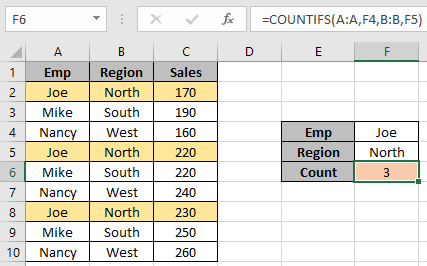In this article, we will learn How to Count values meeting multiple criteria in different columns in Excel.

Scenario:

In Excel, we sometimes need to count the values that lay between the two given values. Example if we need to find the count of certain IDs where the number corresponding to ID value must lay between given ranges. Let's understand how to use the excel function to get the Criteria inside the formula executed using the operators.

COUNTIFS formula in Excel

Excel provides COUNTIFS function that counts on multiple conditions. Now we will make a formula out of the function. Here we are given some values in a range and specific range as criteria. We need to count the values where the number lay between given criteria.

COUNTIFS formula

Syntax:

 = COUNTIFS (range1, criteria1, [range2], [criteria2],...)

Range1 : first range for criteria1.

Criteria1 : criteria from range1.

Range2 : range for criteria2. Optional.

Criteria1 : criteria from range2. Optional.

SUMPRODUCT formula in Excel

For this problem, we will be required to use the SUMPRODUCT function. Now we will make a formula out of the function. Here we are given two ranges of data and we need to count the rows that meet 3 criterias. SUMPRODUCT function returns the SUM of corresponding TRUE values (as 1) and ignores values corresponding to FALSE values (as 0) in the returned array

SUMPRODUCT formula

 = SUMPRODUCT ( ( rng_1 op_1 crit_1 ) + 0 , ( rng_2 op_2 crit_1 ) + 0 , rng_2 op_2 crit_1 ) + 0 )

rng : range to look for

crit : criteria to apply

op : criteria operator, condition given as operator between range & criteria

+0 : converts boolean values to binary(0 & 1).

Example :

All of these might be confusing to understand. Let's understand how to use the function using an example. Here we have a table_array and We need to find the count of the values in the list having 2 conditions. Both criteria needs to match using one single formula in F6 cell.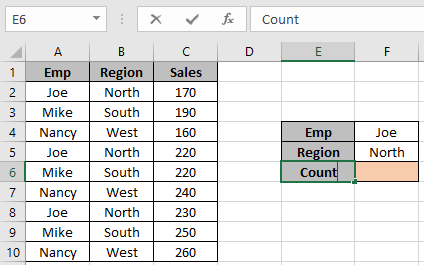We need to find the row count using the below formula

Use the formula:

 = COUNTIFS ( A:A, F4, B:B, F5)

Explanation:

The function matches value in F4 ( Joe ) with column A. And then matches the value in F5 ( North ) with column B.

So the function returns the count of rows having both conditions.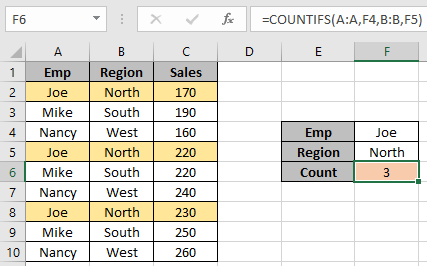As you can see the formula returns the value for two given conditions.

Another Example:

We have this data for our next COUNTIFS example.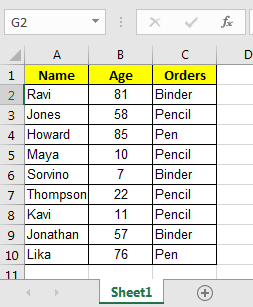And, we have these two queries to answer.So for the first query we have two conditions from one single column, Age.

To answer the query, Count People whose age is Between 50-80 all inclusive, write this COUNTIFS formula.

 =COUNTIFS(B2:B10,">=50",B2:B10,"<=80")

Note that, we have the same criteria range B2:B10  for both conditions. Excel has no objection to the use of the same criteria ranges. With the picked data output will be 3.

Briefing

Simple, the k function will first look for all values in range B2:B10 that will be {81,58,85,57,76}. Then in the {81,58,85,57,76} list it will look at all values that are less than or equal to 80 and that will be {58,57,76}. And that is 3. Now in the second query in which I need to Count People who ordered Pencil and age is less than 15. We have to count on multiple criteria and different columns.

So  two answers to this query write this COUNTIFS formula.

Use the formula

 =COUNTIFS(C2:C10,"Pencil",B2:B10,"<15")

Note that we used two different columns for our condition.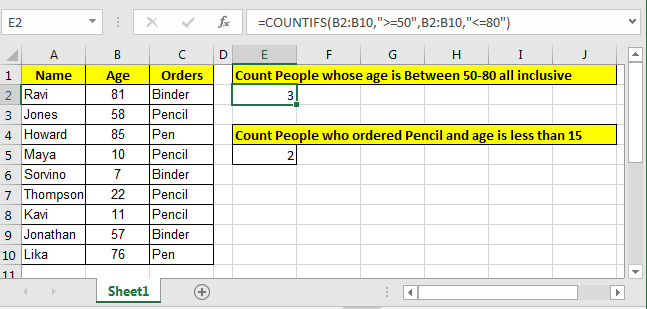Well this is the  classic way of counting in excel for two or more criteria. But there is an alternative way to count if two criteria match.

You can also use the alternative function inplace of the COUNTIFS function. Learn How to use the SUMPRODUCT function in Excel

Here are all the observational notes using the formula in Excel
Notes :

1. The formula only works with numbers and text both.
2. The formula ignores text value while comparing numbers and ignores numbers when match text values.
3. Operators like equals to ( = ), less than equal to ( <= ), greater than ( > ) or not equals to ( <> ) can be performed within a function applied with number only.

Hope this article about How to Count values meeting multiple criteria in different columns in Excel is explanatory. Find more articles on calculating values and related Excel formulas here. If you liked our blogs, share it with your friends on Facebook. And also you can follow us on Twitter and Facebook. We would love to hear from you, do let us know how we can improve, complement or innovate our work and make it better for you. Write to us at info@exceltip.com.

Related Articles :

How to use the SUMPRODUCT function in Excel: Returns the SUM after multiplication of values in multiple arrays in excel.

COUNTIFS with Dynamic Criteria Range : Count cells dependent on other cell values in Excel.

COUNTIFS Two Criteria Match : Count cells matching two different criteria on list in excel.

COUNTIFS With OR For Multiple Criteria : Count cells having multiple criteria match using the OR function.

The COUNTIFS Function in Excel : Count cells dependent on other cell values.

How to Use Countif in VBA in Microsoft Excel : Count cells using Visual Basic for Applications code.

How to use wildcards in excel : Count cells matching phrases using the wildcards in excel

Popular Articles :